# Scale Scale Factor Scale Drawings Scale Scale Factor

• Slides: 18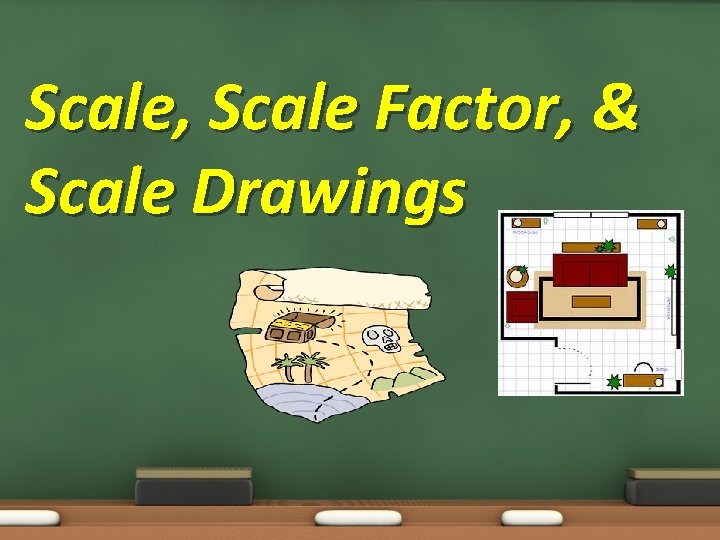Scale, Scale Factor, & Scale DrawingsScale, Scale Factor, & Scale Drawings Objective: 7. 2. 01 & 7. 3. 03 Essential Question: How can I use a scale factor to understand distances on a map or the size of a house or object?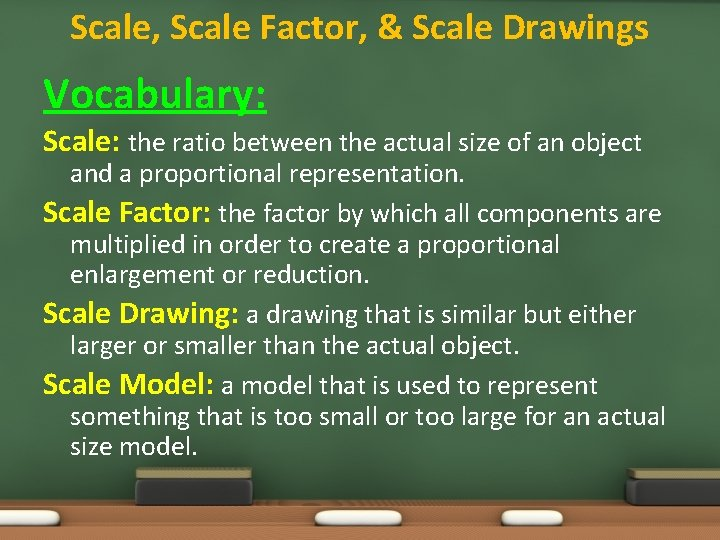Scale, Scale Factor, & Scale Drawings Vocabulary: Scale: the ratio between the actual size of an object and a proportional representation. Scale Factor: the factor by which all components are multiplied in order to create a proportional enlargement or reduction. Scale Drawing: a drawing that is similar but either larger or smaller than the actual object. Scale Model: a model that is used to represent something that is too small or too large for an actual size model.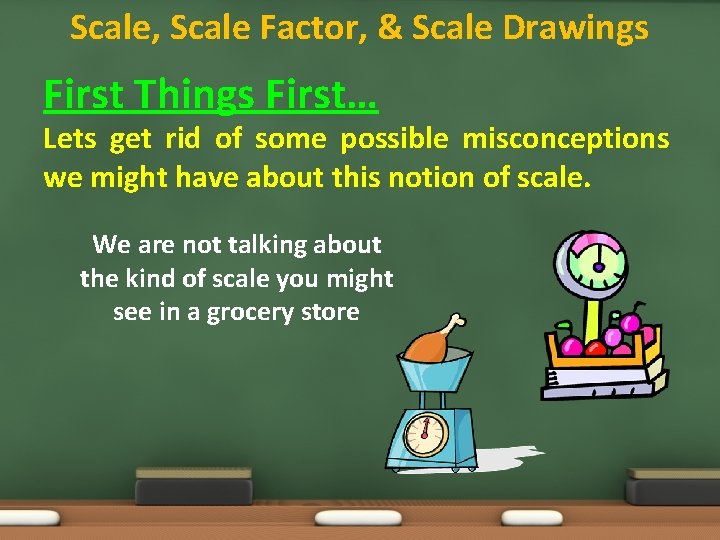Scale, Scale Factor, & Scale Drawings First Things First… Lets get rid of some possible misconceptions we might have about this notion of scale. We are not talking about the kind of scale you might see in a grocery storeScale, Scale Factor, & Scale Drawings First Things First… Lets get rid of some possible misconceptions we might have about this notion of scale. We are also not talking about the kind of scale you get on at a doctors office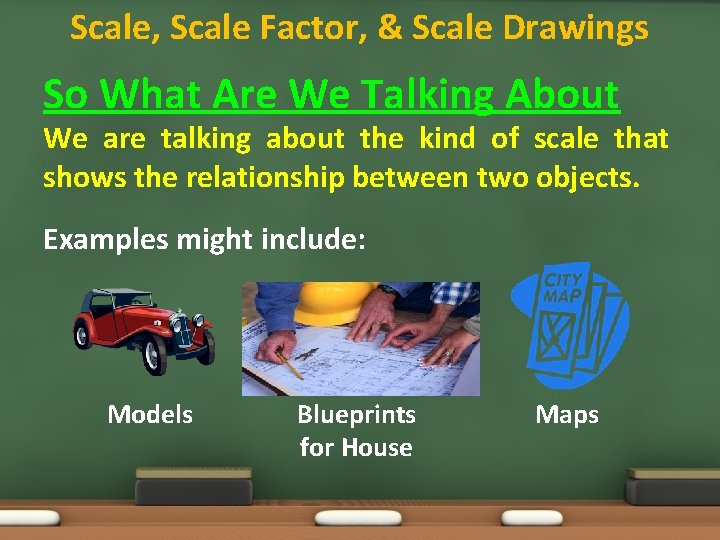Scale, Scale Factor, & Scale Drawings So What Are We Talking About We are talking about the kind of scale that shows the relationship between two objects. Examples might include: Models Blueprints for House MapsScale, Scale Factor, & Scale Drawings Where Will I See This Some careers or jobs that utilize the math concept of scale include: Mapmakers (Cartographers) Model Makers Contractors Doctors Engineers Construction Workers Artists Truck Drivers Architects Pilots Fashion/Clothes Designers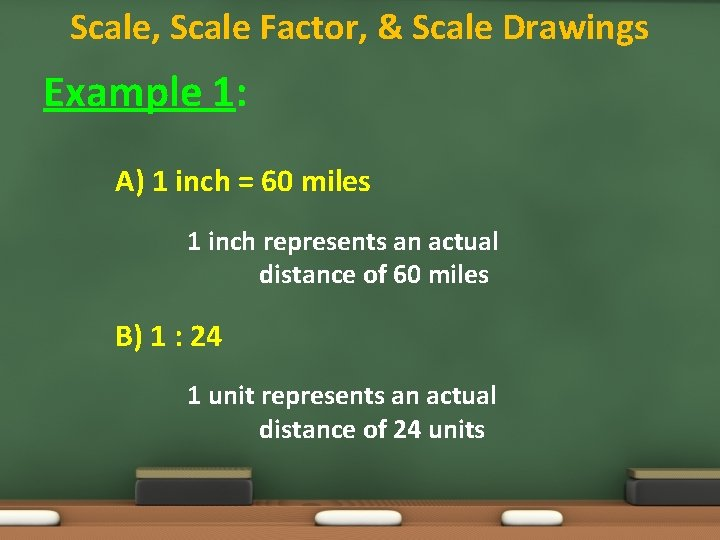Scale, Scale Factor, & Scale Drawings Example 1: A) 1 inch = 60 miles 1 inch represents an actual distance of 60 miles B) 1 : 24 1 unit represents an actual distance of 24 units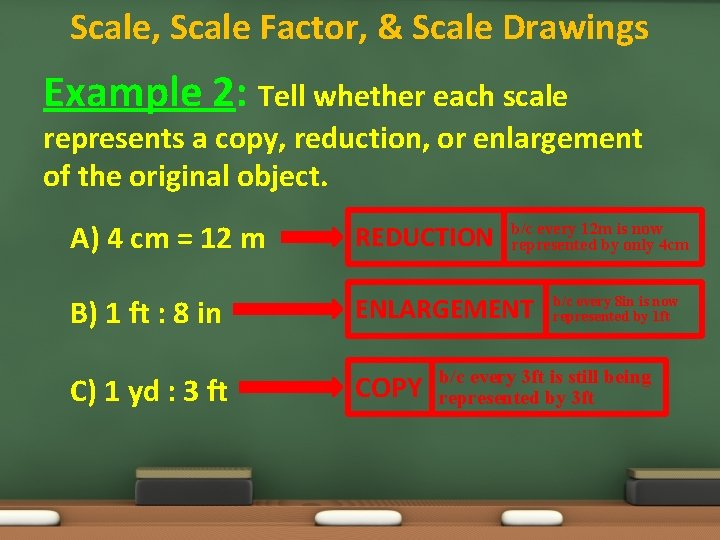Scale, Scale Factor, & Scale Drawings Example 2: Tell whether each scale represents a copy, reduction, or enlargement of the original object. A) 4 cm = 12 m REDUCTION B) 1 ft : 8 in ENLARGEMENT C) 1 yd : 3 ft COPY b/c every 12 m is now represented by only 4 cm b/c every 8 in is now represented by 1 ft b/c every 3 ft is still being represented by 3 ft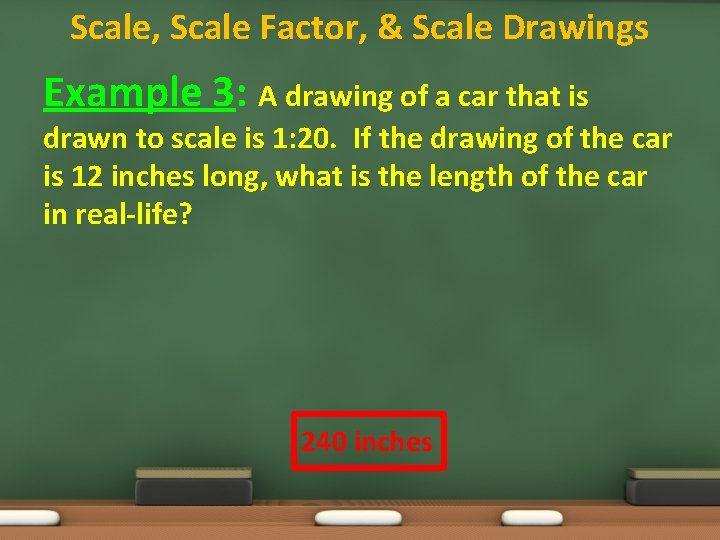Scale, Scale Factor, & Scale Drawings Example 3: A drawing of a car that is drawn to scale is 1: 20. If the drawing of the car is 12 inches long, what is the length of the car in real-life? 240 inchesScale, Scale Factor, & Scale Drawings Example 4: On a map of the United States the scale is 1 inch = 120 miles. A) If the distance from Clinton to Greensboro is 2. 5 inches on the map, how many miles apart are the two cities? 300 milesScale, Scale Factor, & Scale Drawings Example 4: On a map of the United States the scale is 1 inch = 120 miles. B) If the actual distance from Clinton to Wilmington is 60 miles, how far apart should they be on the map? 0. 5 inches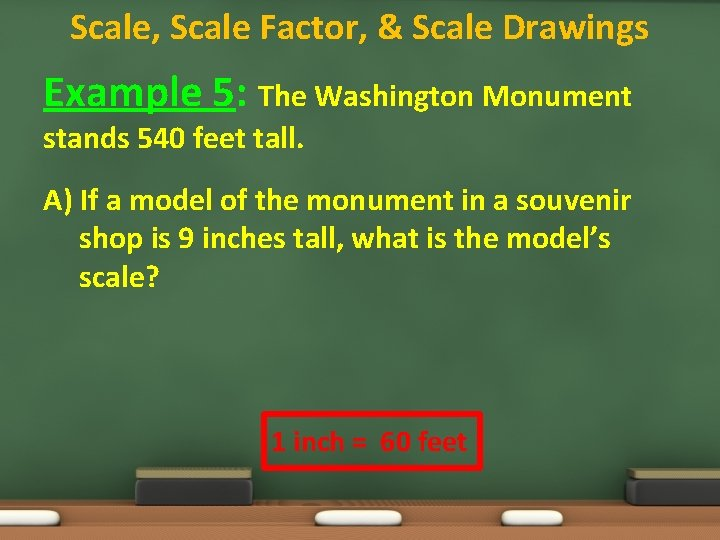Scale, Scale Factor, & Scale Drawings Example 5: The Washington Monument stands 540 feet tall. A) If a model of the monument in a souvenir shop is 9 inches tall, what is the model’s scale? 1 inch = 60 feetScale, Scale Factor, & Scale Drawings Example 5: The Washington Monument stands 540 feet tall. B) What is the scale factor? * scale factor is when both measures have the same unit 1 inch = 720 inches OR 1: 720Scale, Scale Factor, & Scale Drawings Example 6: Below are two similar rectangles. Find the scale factor of the larger rectangle to the smaller rectangle. 24 cm 12 cm 20 cm 10 cm 6: 5Scale, Scale Factor, & Scale Drawings Real World: Your Social Studies teacher has asked you to draw a map of North Carolina. You decide that the scale you will use is 1 inch = 30 miles. The actual distance from Charlotte to Raleigh is 144 miles. How far apart should the cities be on your map? 4. 8 inches apartScale, Scale Factor, & Scale Drawings Real World: The distance from Springfield to Capital City is 1. 4 inches on the map. Find the actual distance. 9. 8 miles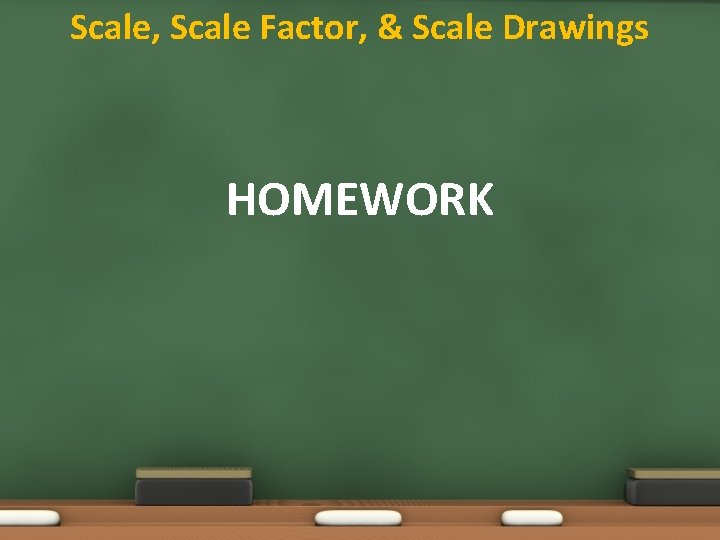Scale, Scale Factor, & Scale Drawings HOMEWORK